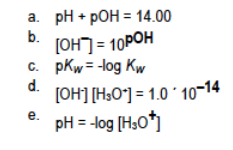# Problem: Which of the following relationships is incorrect? Assume 25° C.

###### FREE Expert Solution
81% (253 ratings)
###### Problem Details

Which of the following relationships is incorrect? Assume 25° C.What scientific concept do you need to know in order to solve this problem?

Our tutors have indicated that to solve this problem you will need to apply the Ka and Kb concept. You can view video lessons to learn Ka and Kb. Or if you need more Ka and Kb practice, you can also practice Ka and Kb practice problems.

What is the difficulty of this problem?

Our tutors rated the difficulty ofWhich of the following relationships is incorrect? Assume 25...as low difficulty.

How long does this problem take to solve?

Our expert Chemistry tutor, Jules took 1 minute and 48 seconds to solve this problem. You can follow their steps in the video explanation above.

What professor is this problem relevant for?

Based on our data, we think this problem is relevant for Professor Maxwell's class at UCF.